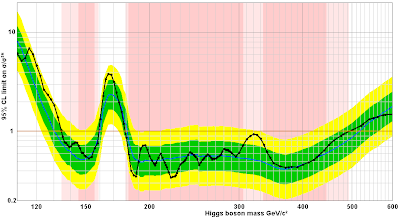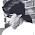## Tuesday, June 12, 2012

### Speculating on CMS hints of $$320\GeV$$ MSSM Higgs

TRF has considered that $$125\GeV$$ Higgs to be a sure thing since December 14th, 2011. New signals appear every day as experimental physicists say that they're indeed closer to the most official discovery you may get.

But I am convinced that even those people who say that they remain "open-minded" won't really be thrilled once the signal reaches the "official discovery" threshold. A convention may say that 4.5 sigma (the highest confidence level obtained from the 2011 combinations) and 6 sigma (which you may get now) are qualitatively different signals – only the latter is a discovery – but everyone correctly feels that the difference between them is relatively small.

In the absence of clear enough deviations of the published LHC data from the Standard Model, we may focus on the Higgs charts. If you play with the viXra combo Higgs java applet, you may try to search for other excesses besides the obvious $$125\GeV$$ line.

Luciano Maiani, a senior Italian physicist – who is famous for having stopped the LEP collider in 2000 when it was promising to discover a $$115\GeV$$ Higgs boson (it seems likely now that there's nothing over there and $$125\GeV$$ seems too far, so Nature has endorsed Maiani's decision now) – and his two collaborators fell in love with a rather small 2-sigma excess seen especially by the CMS detector especially in the $$ZZ$$ and perhaps $$\gamma\gamma$$ channel.If you go to Phil Gibbs' combo applet, you may switch the experiment to "custom" and pick CMS; and switch the channel to "custom" and pick $$H\to ZZ$$. Where will you see the greatest peak? Yes, it will be near $$320\GeV$$, about 2 standard deviations above the mean.

This value of the mass is of course excluded as the mass of the (only) Higgs boson of the Standard Model, but much less safely excluded than the adjacent masses. More importantly, it could be another Higgs if supersymmetry is right and if its Higgs sector is not decoupled (note that Kane et al., for example, predict that only the single MSSM Higgs is light enough). Recall that supersymmetry implies that the God particle has five faces.

So Maiani, together with Polosa and Riquer, boldly assume that the extra excess near $$320\GeV$$ is a sign of the heavier CP-even neutral Higgs boson, $$H$$, and they try to check whether the emerging picture with the $$h,H$$ Higgs bosons at $$125$$ and $$320\GeV$$ seems sensible and consistent with the data.
Probing Minimal Supersymmetry at the LHC with the Higgs Boson Masses (arXiv)
In this freshly improved and updated February 2012 paper, they calculate various relationships between the masses given the assumption and the cross sections and their answer is that the hypothesis does seem sensible. What are the other supersymmetric parameters they're able to predict from their assumptions?$\eq{ \tan\beta&\approx 2\\ M_H &= M_{H^\pm}=M_A= 320\GeV\\ \sqrt{M_{\tilde t_R} M_{\tilde t_L}} &\approx 3.9\TeV }$ You see that $$\tan\beta$$ is relatively small in this scenario and all the four other Higgses clump near $$320\GeV$$. They also say that the number of $$H$$ events decaying to $$ZZ$$ should be about 3 times smaller than in the Standard Model. The charged and CP-odd Higgses would have clear signals while decaying to bottom quark pairs and/or $$t\bar b$$. The "geometric average" stop squark mass would be near $$4\TeV$$.

The heavy $$H$$ Higgs boson is created and decays to two photons at a rate that is 4 times lower than the figure for the Standard Model.

I think that one additional number they mention is rather worrisome for their point in the parameter space and, in fact, MSSM in general. The $$\gamma\gamma$$ decay channel of the light Higgs is predicted to be 20% weaker than in the Standard Model; preliminary inaccurate data suggest that it could, on the contrary, be up to 100% greater.

Of course, all these speculations are experimentally supported by a 2-sigma bump only so it may easily go away and it probably will. But once again, there's no law that would say that all bumps will go away and there will never be any new physics anymore...

1."there's no law that would say that all bumps will go away and there will never be any new physics anymore... "

The second part of this does NOT follow from the first - the bumps could go away, but it would certainly take some "physics" to make that happen.

I did not try applet, I do not know if it is tainted.

(Like AGE applets. Input amount of CO2 that will be added to atmosphere, find out how much Earth's temperature will "rise")

2.Thanks for your comment, Brian, but there must be some misunderstanding here.

The two parts of the sentence say exactly the same thing. If there's a "bump [deviation from the Standard Model charts/predictions] that doesn't go away", it's exactly the same thing as "new physics".

If there's no bump that is more than noise that goes away, it means that the Standard Model is OK and there's no visible new physics. And vice versa. If there's a bump that gets greater as you collect more data, it proves that the Standard Model is wrong and there are new physical phenomena.

I don't understand your "tainted applet" comment. The applet is a straightforward program to sum up - and do other simple enough operations - out of the publicly known data about the number of events of various kinds at the LHC.

Phil's formulae for the combinations could be a bit less sophisticated and precise than the "official ones" but they have turned out to be almost indistinguishable visually - and one may be sure that if there are big bumps in one source, there will also be bumps in the other source.

Cheers
LM